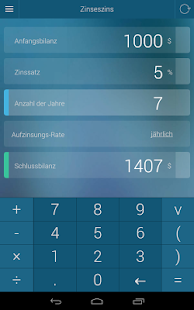Everyday calculations
* simple percentage calculator (5 percent of 40 is 2)
* percentage increase/decrease (5 percent decrease from 40 is 38)
* tip calculator
* discount calculator
* convert fractions to percentages (5 / 20 is the same as 25 percent)

* markup calculator
* profit margin calculator
* VAT
* Sales tax
* a powerful trader’s calculator (VAT or sales tax, net cost, gross cost, markup/profit margin, my net price, my gross price and profit all in one calculator)
* compound interest
* loan payment
* cumulative growth
* Compound Annual Growth Rate (CAGR)
* inflation
* doubling time (rule of 72)

Percentage Calculator’s strongest feature is that any value can either be a source or result of a calculation – just enter the values you know and it will tell you the remaining ones!

Percentage Calculator is useful in many real-life situations:
* school (mathematics, statistics, algebra)
* business and finance (markup, profit margin, profit, loan payments, cumulative growth, inflation, doubling time, investment return rate, loan interest rate, company profits changes). Sales people love the markup and profit calculator!
* shopping (discounts, comparison of two products that differ in quantity)
* tipping
* cooking (ingredients are often shown in percentages)
* health (body mass index, fat percentage in food)

What’s New:
No Changelog

Screenshots

•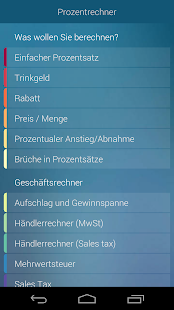•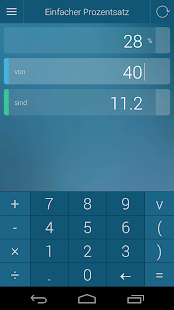•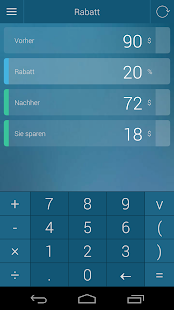•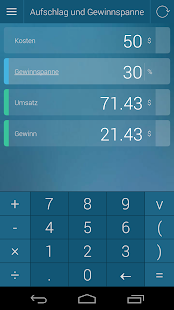••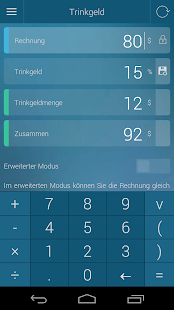•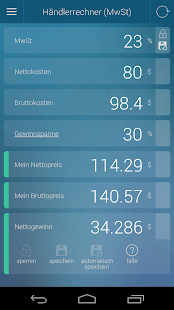•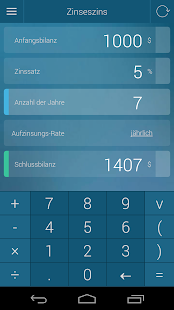•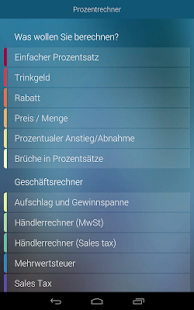•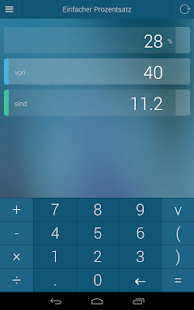•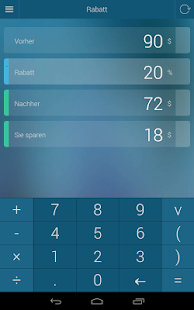•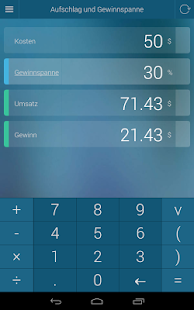•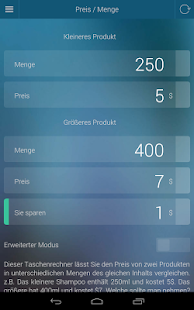•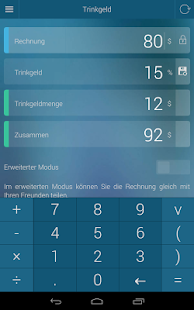•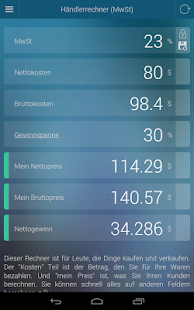•